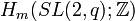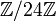# Group cohomology of special linear group of degree two over a finite field

## Contents

This article gives specific information, namely, group cohomology, about a family of groups, namely: special linear group of degree two. This article restricts attention to the case where the underlying ring is a finite field.
View group cohomology of group families | View other specific information about special linear group of degree two | View other specific information about group families for rings of the type finite field

This article describes the group cohomology of the special linear group of degree two over a finite field. The order (size) of the field is$q$, and the characteristic prime is$p$.$q$ is a power of$p$. We denote the group as$SL(2,q)$ or$SL_2(q)$.

Each$SL(2,q)$ is a finite group with periodic cohomology, and the period is 4. We can confirm this from the subgroup structure of special linear group of degree two over a finite field: all the Sylow subgroups are either cyclic groups or generalized quaternion groups.

The cases$q = 2$ (see group cohomology of symmetric group:S3) and$q = 3$ (see group cohomology of special linear group:SL(2,3)) are somewhat anomalous.

## Particular cases$q$ (field size)$p$ (underlying prime, field characteristic) Case for$q$ Group$SL(2,q)$ Order of the group ($q^3 - q$) Group cohomology page
2 2 even symmetric group:S3 6 group cohomology of symmetric group:S3
3 3 odd special linear group:SL(2,3) 24 group cohomology of special linear group:SL(2,3)
4 2 even alternating group:A5 60 group cohomology of alternating group:A5
5 5 odd special linear group:SL(2,5) 120 group cohomology of special linear group:SL(2,5)
7 7 odd special linear group:SL(2,7) 336 group cohomology of special linear group:SL(2,7)
8 2 even projective special linear group:PSL(2,8) 504 group cohomology of projective special linear group:PSL(2,8)
9 3 odd special linear group:SL(2,9) 720 group cohomology of special linear group:SL(2,9)

## Homology groups for trivial group action

FACTS TO CHECK AGAINST (homology group for trivial group action):
First homology group: first homology group for trivial group action equals tensor product with abelianization
Second homology group: formula for second homology group for trivial group action in terms of Schur multiplier and abelianization|Hopf's formula for Schur multiplier
General: universal coefficients theorem for group homology|homology group for trivial group action commutes with direct product in second coordinate|Kunneth formula for group homology

### Over the integers

For any$m > 0$ the group$H_m(SL(2,q);\mathbb{Z})$ form a periodic sequence, i.e., the group is a finite group with periodic cohomology. Note that$H_0(SL(2,q);\mathbb{Z}) = \mathbb{Z}$ is anomalous.

Congruence class of$m$ mod 4$H_m(SL(2,q);\mathbb{Z})$ for$q = 2$$H_m(SL(2,q);\mathbb{Z})$ for$q = 3$
1$\mathbb{Z}/2\mathbb{Z}$$\mathbb{Z}/3\mathbb{Z}$
2 0 0
3$\mathbb{Z}/6\mathbb{Z}$$\mathbb{Z}/24\mathbb{Z}$
0 0 0

For$q > 3$,$H_1(SL(2,q); \mathbb{Z}) = H_2(SL(2,q); \mathbb{Z}) = 0$.$H_3$ exhibits interesting behavior.